GeoConstructix – SCOPES Digital Fabrication

### Lesson Details

Subjects
Age Ranges
Fab Tools
Topic Tags
Author

You need to login or register to bookmark/favorite this content.

### Author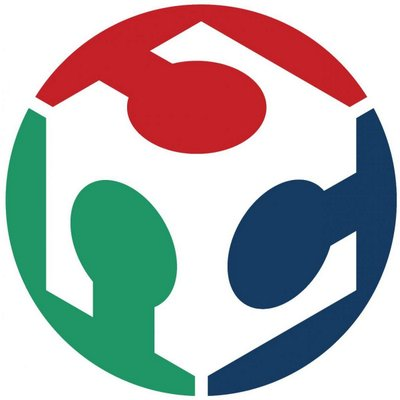Fab Foundation
Maker
Our mission is to provide access to the tools, the knowledge and the financial means to educate, innovate and invent using technology and digital fabrication to allow anyone to make (almost) anything, and thereby creating opportunities to improve lives and… Read More

### Summary

The primary focus of this lesson is on the inter-relationship between geometry and fabrication. GeoConstructix adapts digital fabrication concepts by focusing on the inter-relationship between geometry and Computer-aided design and manufacturing (CAD/CAM) techniques. The lesson’s scaffolding structure allows students to work with a series of successive methods-based exercises that rely on both digital modeling and prototypes. Students “learn by doing.” By the end of this lesson, students will learn the fundamentals of geometry and fabrication techniques with increasing levels of complexity. This is an introductory lesson in digital fabrication.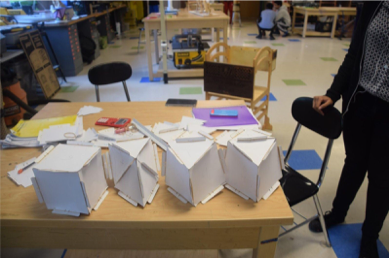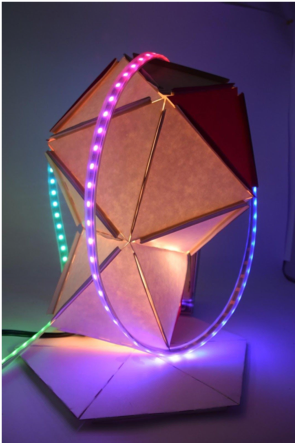### What You'll Need

Key Vocabulary / Terms

Geometry
Area
Volume
Symmetry
Angles
Fundamental shapes
Face
Edge
Vertex
Cube
Tetrahedron
GeoConstructix

Key Formulas

Area of Triangle = ½ base * height
Area of a Square = base * height
Cube Volume = base * height * depth
Tetrahedron = a3/ (6√2) ; a = length of edge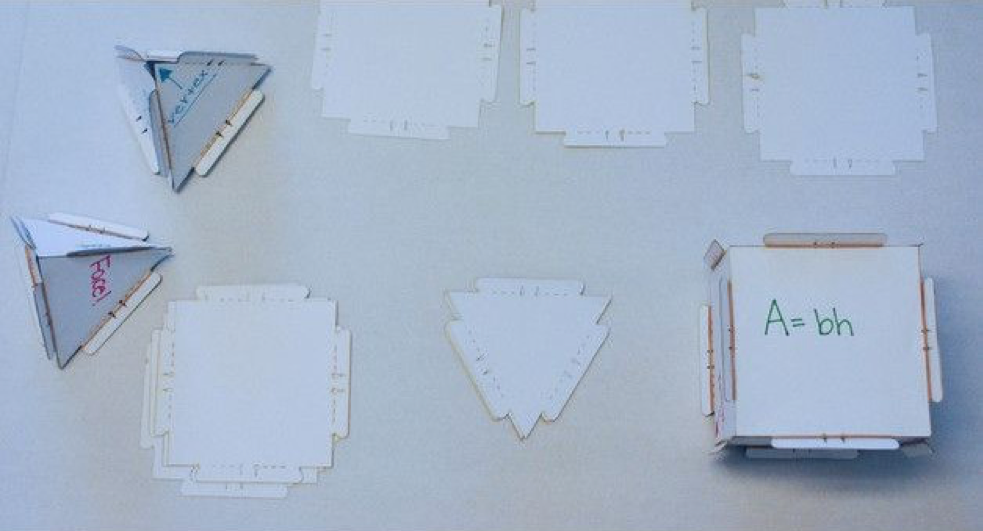Materials List

110 lb Cardstock
Laser cutter
CorelDraw (or similar 2D vector graphics software)
Rubber bands

Design Files Developed:

GeoConstructix – Grade 7 – Voluminous Sculptures – Student

## The Instructions

Map of Lesson

1. Step 1 – Use GeoConstructix for identifying geometry concepts to create non-digital prototypes
2. Step 2 – Build Your Own Sculpture

Before the lesson:

• Teacher will precut the GeoConstructix Each piece requires approximately 1.5 minutes.
• If desired, the lesson can be adapted to allow for the students to cut their own components out.

Step One: Identify geometry concepts to create non-digital prototypes (25 Minutes)

Guided Instruction: Students will gain an understanding of the relationship between fabrication process and assembly without technical aspects of digital modeling. Teacher will guide students through an activity in which they use laser cut 2D shapes to construct various 3D objects and learn about geometric shapes.

Essential Question: Why is it important to understand the characteristics and distinctions of two-and-three-dimensional figures?

Make sure students have the following supplies before beginning lesson plan:

• 6x square GeoConstructix
• 4x triangle GeoConstructix
• 16x rubber bands
1. Bell Ringer (15 Minutes): Provide students with a working understanding of Area vs. VolumeOn classroom smartboard, have students watch the following Khan Academy tutorials:

Intro to area and unit square:

1. https://www.khanacademy.org/math/basic-geo/basic-geo-area-and-perimeter/basic-geo-unit-square s-area/v/introduction-to-area-and-unit-squaresMeasuring volume as area times length:
https://www.khanacademy.org/math/basic-geo/basic-geo-volume-sa/volume-rect-prism/v/measuring-volume-as-area-times-lengthRemind students of definitions:Definition: Area is the size of a surface of a 2-dimensional object such as a triangle or square. It has units of square inches (in2)Definition: Volume is the amount of space inside a solid 3-dimensional object such as a cube or sphere. Volume has dimensions of cubic inches (in3)
2. Transition (1 minute): Instruct students that they are to keep a definition list in their notebook; and each time they encounter new geometry vocabulary, add the term to their list.Illustrate each definition with a simple sketch.Tell students that the following fabrication geometry prototypes will be explored through this initial exercise.
– Building a Cube
– Building a TetrahedronTell students that two important reference equations are:Area of Triangle = ½ base * height
Area of a Square = base * height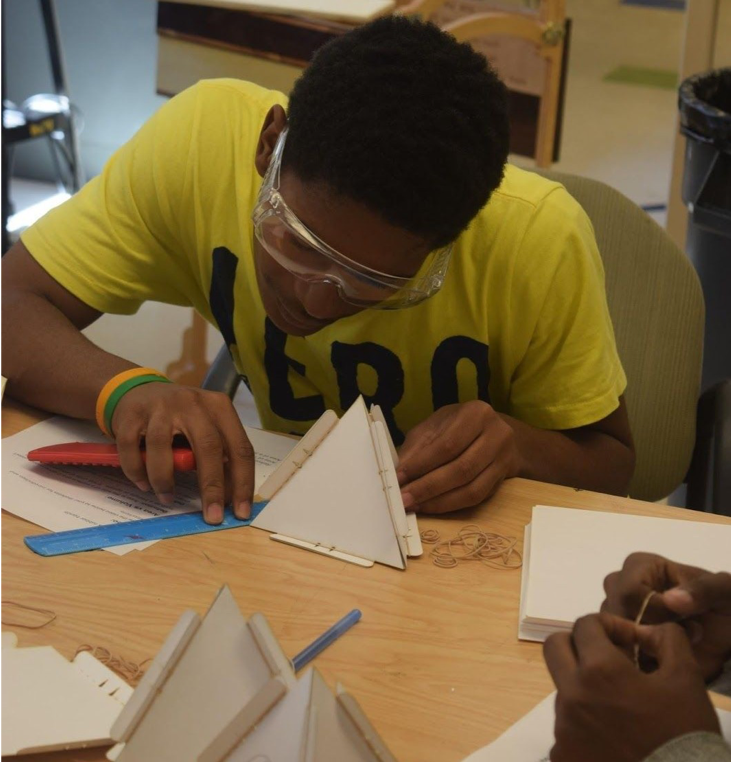3. Building a cube (3 minutes):
1. Use 6 square GeoConstructix to build a cube using rubber bands as shown below.
• Fold all the flaps along the dashed line
• Connect edges with rubber bands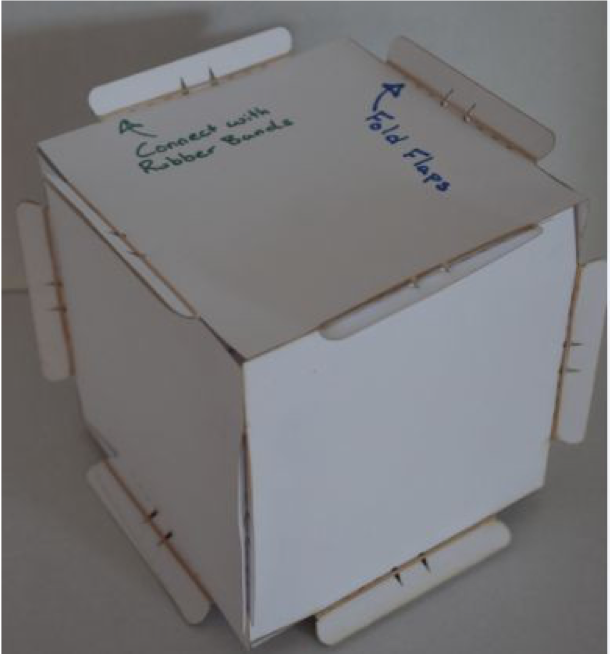2. How many of the following are in your cube?
1. Faces:
2. Vertices:
3. Edges: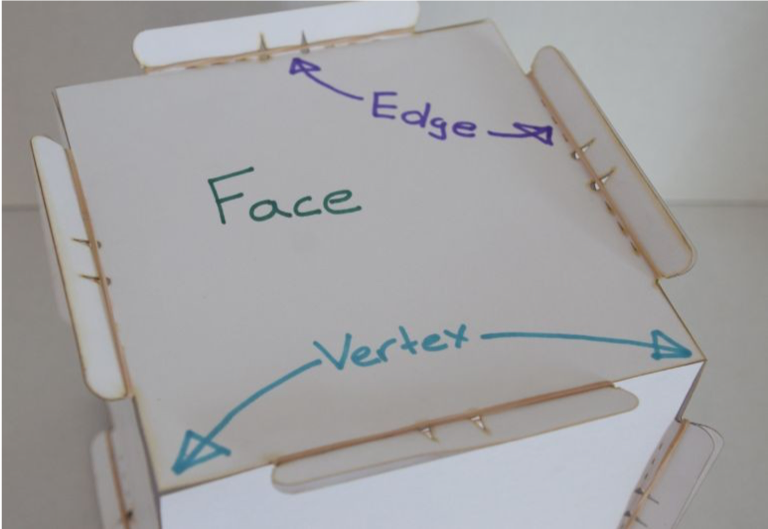3. How many edges come together at each vertex?
4. Measure the length of the edges to calculate the volume?Cube Volume = base * height * depthVCube = b * h * d
4. Building a Tetrahedron (3 minutes)
A tetrahedron is a 4 sided platonic solid, made of triangles.

• Use 4 GeoConstructix triangles to build the shape
• How many edges come together at each vertex?Surface area is the total area of a 3-dimensional object. Find the area of each face and add it together to find the surface area.
• What is the surface area of the tetrahedron?
• What is the volume of the tetrahedron?
•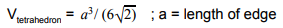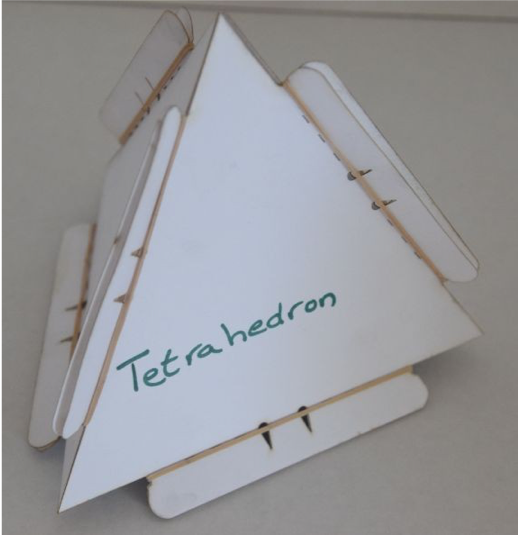5.  Connecting Shapes (3 minutes)
Connect the cube and the tetrahedron to make the following structure.What is the total number of:
Edges:
Vertices:
Faces:What is the total surface area of the new sculpture?
6. Exit Ticket (10 Minutes)
1. Describe the difference between area and volume?
2. What is the area of the triangle GeoConstructix?
3. What is the area of the square GeoConstructix?
4. What is the unit of area?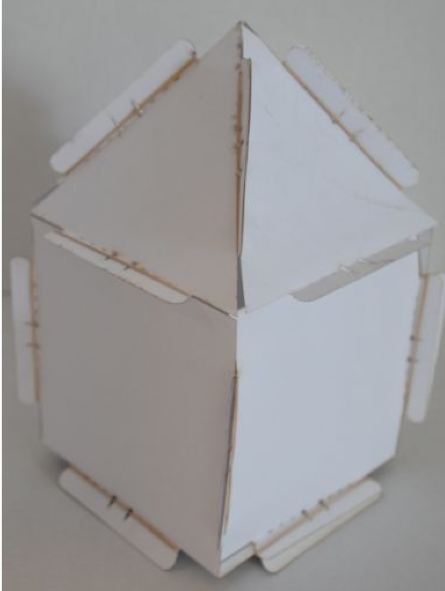Step 2: Build Your Own Sculpture

Essential Question: In what ways are geometric figures used in real life?

Warm-up (10 Minutes): Describe the the fundamentals of laser cutting:

• What computer program is used to control the laser?
• What does it mean to vector?
• What settings must you change if you want to vector a line?
• What does it mean to raster?
• Describe the required steps to focus the laser?

Question for students:

• Has anyone seen a laser cutter before?
• Has anyone used a laser cutter before?
• What are some things you might cut with a laser?
• Who knows the steps in the engineering design process?: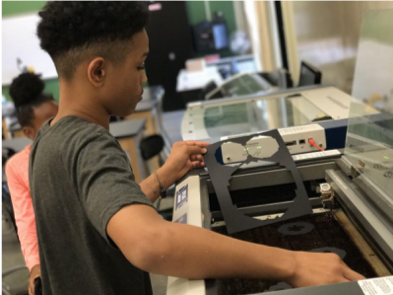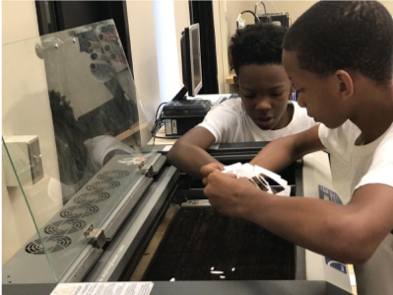Making of Shapes: (50 Minutes)

1. Begin by explaining and modeling: In whole class setting review how to use the laser cutting machine.
2. Guide the students to design their own sculptures, using the basic shapes from Step 1 as foundations or jumping off points, and adding other embellishments such etched designs, LED electronics, and other craft materials.
• Say:
• To complete your own creative sculpture get additional pieces from the supply bins. Be sure to save supplies for other groups.
• Use the additional pieces to build your own unique geometric sculpture. Work with your team to connect all of your pieces in an interesting pattern.
• Show examples for inspiration.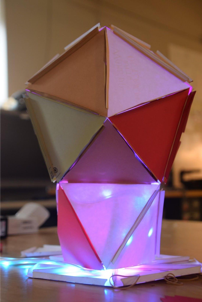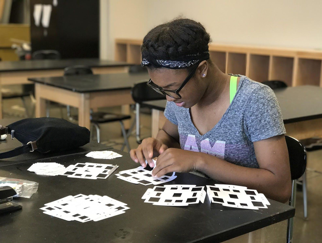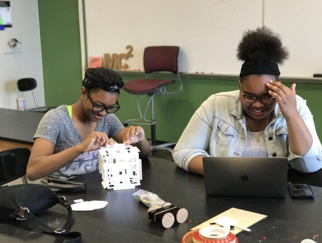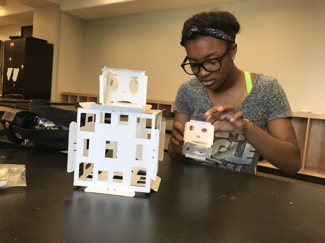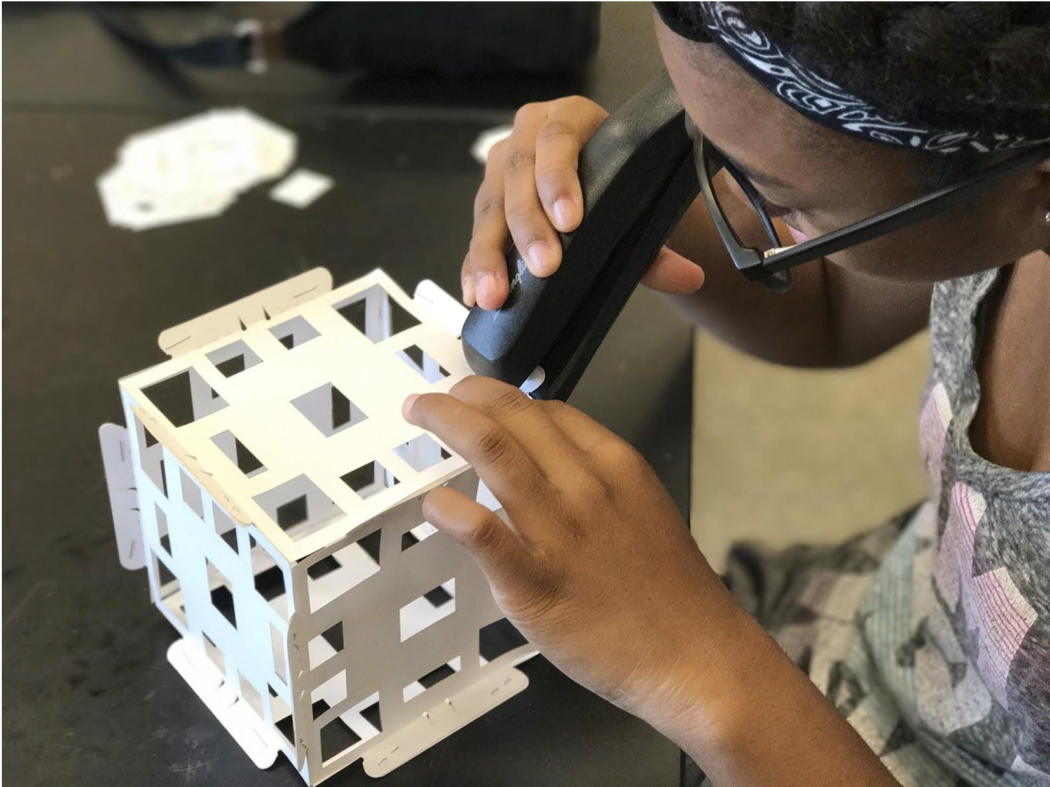(From Fab Testing in Cleveland (Andrea Fields))

## Standards

Common Core Mathematical Practices

MP.1- Make sense of problems and persevere in solving them.

MP.2 -Reason abstractly and quantitatively.

MP.3- Construct viable arguments and critique the reasoning of others.

MP.4- Model with mathematics.

MP.5-Use appropriate tools strategically. MP.6- Attend to precision.

MP.7- Look for and make use of structure.

MP.8- Look for and express regularity in repeated reasoning.

Common Core Mathematics Standards

5.G.A.1. Use a pair of perpendicular number lines, called axes, to define a coordinate system, with the intersection of the lines (the origin) arranged to coincide with the 0 on each line and a given point in the plane located by using an ordered pair of numbers, called its coordinates.

5.NBT.5:Fluently multiply multi-digit whole numbers using standard algorithm.

6.RP.1: Understand the concept of a ratio and use ratio language to describe a ratio relationship between two quantities.

6.RP.3-b: Solve unit rate problems including those involving pricing and constant speed.

6.RP3-d: Use ratio reasoning to convert measurement units; manipulate and transform units appropriately when multiplying or dividing.

Digital Fabrication Competencies: I Can Statements

• (S.1) Safety: I can safely conduct myself in a Fab Lab, observe operations and follow general safety protocols under guidance from an instructor.
• (DP.3) Design Process: I can create analog models (e.g. sketches, small physical models, ) to facilitate a design process.
• (DP.4) Design Process: I can record and share my ideas during a design process to document the learning process (e.g. journal writing, group reviews, etc.).
• (DP.5) Design Process: I can work with a group to follow multiple common design process steps (e.g. defining the user, brainstorming, prototyping, iterating, etc.).
• (F.1) Fabrication: I can assemble an object using prefabricated components.
• (SC.1) Sustainability and Commerce: I use scrap and renewable resources like cardboard first, before using higher cost materials. I understand the cost of various raw materials in the FabLab.
• (CT.1) Critical Thinking: I can use information in a Fab Lab (or through use of digital fabrication tools and processes) to answer questions about the design process.
• (Q.2) Questioning: I can formulate questions that reveal important aspects of design process including problems and challenges.
• (PS.3) Proposed Solution: I can propose alternative solutions to a design problem through iterations and determine their utility through execution.